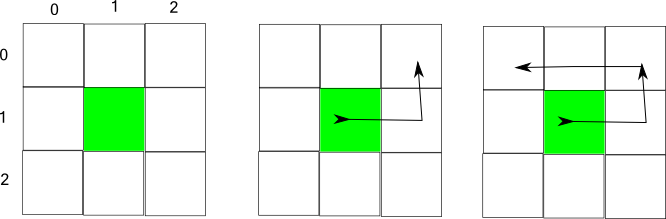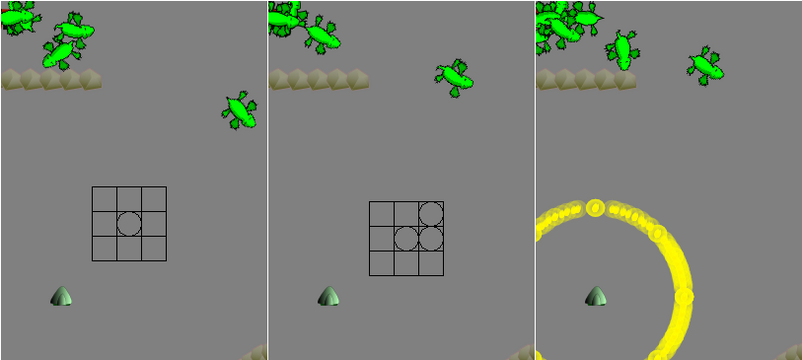posted on: 2011-05-23 13:03:06
I have implemented a simple gesture algorithm.

>Here is my simple gesture algorithm for a video game, Rainy Day Wizards that I have been writing. The result is when you right-click to cast a spell, you can use a gesture to cast more powerful spells. I have only implemented one spell, but the engine is in place to implement more.

The idea is there there are a number of boxes, and as you drag the mouse from box to box, you accumulate points in the path. When the mouse is released the path is compared to existing paths.I pack all of the coordinates into an integer so that I can use a switch statement at the end.

On a right click I initialize the path:

```/**
* Starts  a new path for a gesture.
* @param p starting point.
*/
public void begin(Point2D p){
gesturing = true;
origin = p;

//packs xy coordinates, first point is always 1,1
last = (1<<2) + 1;
count = 0;

//path will accumulate all of the points.
path=last;
count++;

}

```

By using an integer I have limited the maximum number of my boxs to 4x4 and the maximum number of points in my gesture to 8, but that is sufficient for now.

The next step is updating the path. Note that a majority of this next step is just making certain the point is in a valid new box.

```/**
* Finds the relative position of the current point, and places it
* into a gesture box.  Each box is (xdex<<2) + ydex
*
* @param p
*/
public void update(Point2D p){
//too many gesture points return.
if(count>=8) return;

//x index of box
int x_dex = (int)(p.getX() - origin.getX() + 1.5* BOX_SIZE);
x_dex = x_dex/ BOX_SIZE;

//only the closest boxes are valid
if(x_dex<0||x_dex>2) return;

//same for y.
int y_dex = (int)(p.getY() - origin.getY() + 1.5* BOX_SIZE);
y_dex = y_dex/ BOX_SIZE;

if(y_dex<0||y_dex>2) return;

//The current position is within the same box ignore
int now = (x_dex<<2) + y_dex;
if(now==last) return;

last = now;

path = (path<<4) + now;
count++;

}
```

I add new points on the right side of the path because of the 0,0 box. Since I always start with a 1,1 box, the 0,0 box will be a place holder.

Finally, when the path is finished, I switch on the resulting integer.

```public int finish(Point2D p){

gesturing = false;

switch(path){
case RING_OF_FIRE:
return 1;
default:
return 0;
}
}
```

Now here is the nasty part I don't want to talk about too much, creating the 'known paths.'

```final static int RING_OF_FIRE =
(((1<<2) + 1)<<16) +
(((2<<2) + 1)<<12) +
(((2<<2) + 0)<<8) +
(((1<<2) + 0)<<4) +
0;
```

I have a function that does this for a set of coordinates, but I cannot use that function with a switch statement. Here are the results.I have it drawing the path for debugging purposes, but I might give it some better graphics and leave it.